GeeksforGeeks App
Open AppBrowser
Continue

## Related Articles

• NCERT Solutions for Class 11 Maths

# Class 11 NCERT Solutions- Chapter 10 Straight Lines – Exercise 10.1

### Question 1. Draw a quadrilateral in the Cartesian plane, whose vertices are (– 4, 5), (0, 7), (5, – 5), and (– 4, –2). Also, find its area.

Solution:

Let’s consider the quadrilateral ABCD with vertices A (-4,5), B (0,7), C (5.-5) and D (-4,-2).

After plotting the points on the Cartesian plane we get the required quadrilateral :

Construction : Join diagonal AC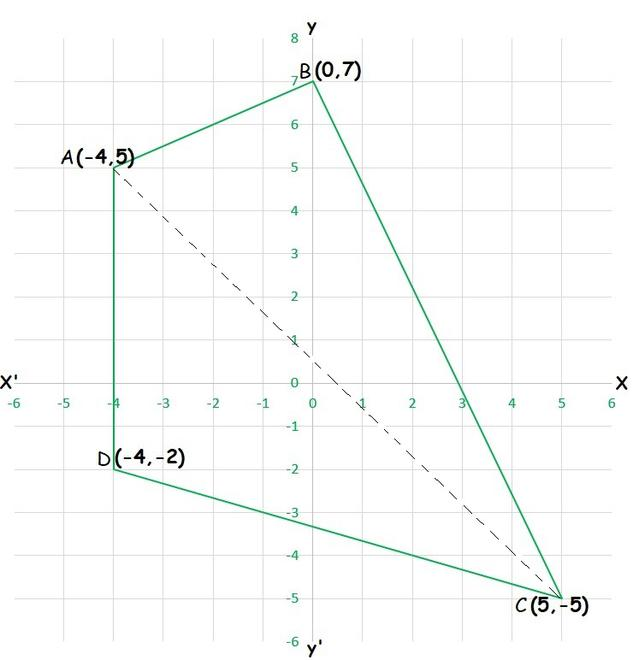Area (ABCD) = area (∆ABC) + area (∆ADC)

Then, area of triangle with vertices (x1,y1), (x2, y2) and (x3,y3) is

= |½ [x1 (y2 – y3) + x2 (y3 – y1) + x3 (y1 – y2)]|

So,

Area (∆ABC)= |½ [-4 (7 + 5) + 0 (-5 – 5) + 5 (5 – 7)] |

= |½ [-4 (12) + 5 (-2)] |

= |½ [-48 -10)] |

= | ½ (58) |

= 29 sq. unit

Area (∆ACD) = |½ [-4 (-5 + 2) + 5 (-2 – 5) + (-4) (5 – (-5))]|

= |½ [-4 (-3) + 5 (-7) – 4 (10)]|

= |½ [-12 + -35 – 40]|

= |½ (-63)|

= 63/2 sq. unit

Area (ABCD) = 29 + 63/2

= 121/2 sq. unit

### Question 2. The base of an equilateral triangle with side 2a lies along the y-axis such that the mid-point of the base is at the origin. Find vertices of the triangle.

Solution:

Let’s consider ∆ABC, which is an equilateral triangle with side 2a.

Where, AB = BC = AC = 2a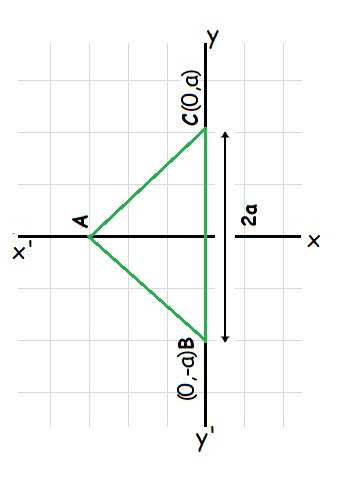As in the figure, we assume BC as base, where origin becomes mid-point of BC. So, we can conclude that

The co-ordinates of point C are (0, a) and that of B are (0,-a).

And as origin is the mid-point, so the line joining vertex and origin lies on x-axis. (x-axix ⊥ y-axis)

(AC)2 = OA2 + OC2        (Pythagoras theorem)

(2a)2= a2 + OA2

4a2 – a2 = OA2

OA2 = 3a2

OA =√3a

Co-ordinates of point A = ± (√3a, 0)

∴ The vertices of the given equilateral triangle are (0, a), (0, -a), (√3a, 0) Or (0, a), (0, -a) and (-√3a, 0)

### (ii) PQ is parallel to the x-axis.

Solution:

(i) When PQ is parallel to the y-axis, then the x-coordinates will be same.

so, x1=x2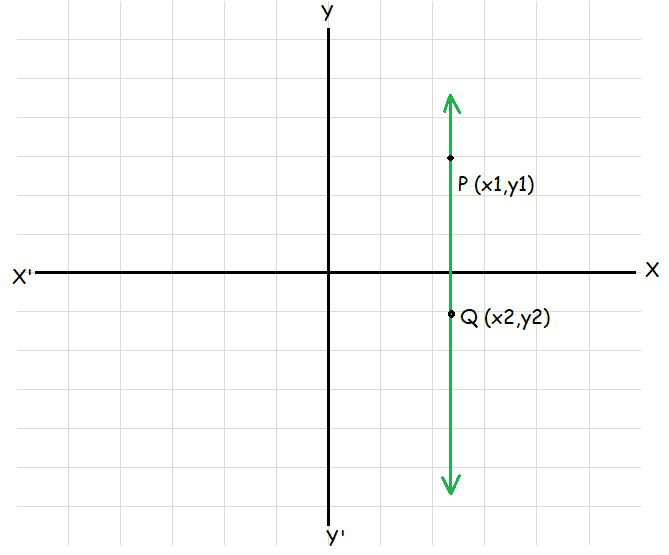Distance between points PQ = √((x1-x2)2 + (y1-y2)2)

= √(y1-y2)                        (As x1=x2)

= |y1-y2|

(ii)

When PQ is parallel to the x-axis, then, the y-coordinates will be same.

so, y1=y2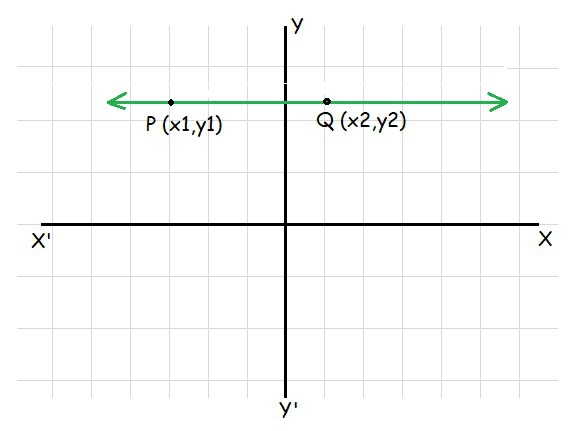Distance between points PQ = √((x1-x2)2 + (y1-y2)2)

= √(x1-x2)2                         (As y1=y2)

= |x1-x2|

### Question 4. Find a point on the x-axis, which is equidistant from the points (7, 6) and (3, 4).

Solution:

As it is mentioned that, these points A and B are equidistant from a point P on x-axis.

Let’s take P as (a, 0).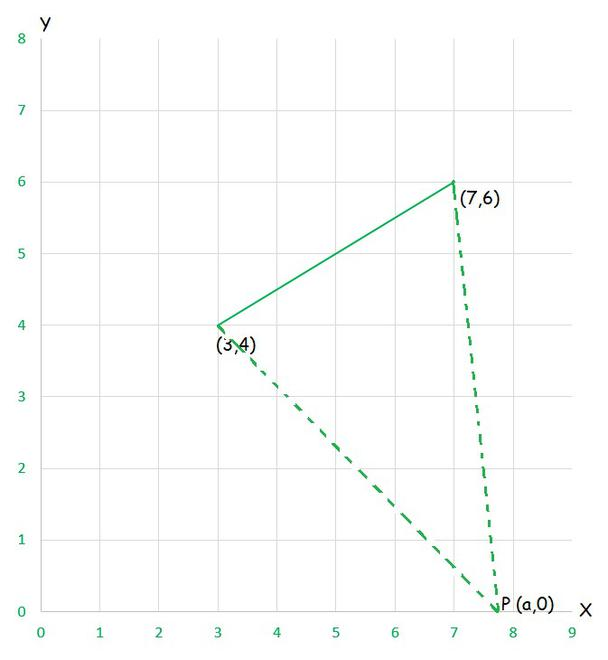Distance AP = Distance BP

Distance between two points (x1,y1) and (x2,y2)= √((x1-x2)2 + (y1-y2)2)

so,

√((3-a)2 + (4-0)2) = √((7-a)2 + (6-0)2)

√((3-a)2 + 16) = √((7-a)2 + 36)

√(9-6a+a2+16) = √(49-14a+a2+36)

Squaring both sides, we get

a2 – 6a + 25 = a2 – 14a + 85

-8a = -60

a = 15/2

Hence, the point on the x-axis, which is equidistant from the points (7, 6) and (3, 4) is (15/2,0)

### Question 5. Find the slope of a line, which passes through the origin, and the mid-point of the line segment joining the points P (0, – 4) and B (8, 0).

Solution:

Let the co-ordinates of mid-point of the line segment joining the points P (0, – 4) and B (8, 0) be (x,y), so

x = (0+8)/2 = 4

y = (-4+0)/2 = -2

Mid point is (4,-2)

As The slope ‘m’ of the line which is passing through the point (x1,y1) and (x2,y2) will be given as

m = (y2 – y1)/(x2 – x1) where, (x2 ≠ x1)

So now, The slope ‘m’ of the line which is passing through the point (4,-2) and origin (0,0) will be

m = (4-0)/(-2-0) = 4/-2 = -1/2

Hence, the required slope is -1/2.

### Question 6. Without using the Pythagoras theorem, show that the points (4, 4), (3, 5), and (–1, –1) are the vertices of a right-angled triangle.

Solution:

As the vertices of the given triangle are (4, 4), (3, 5) and (–1, –1).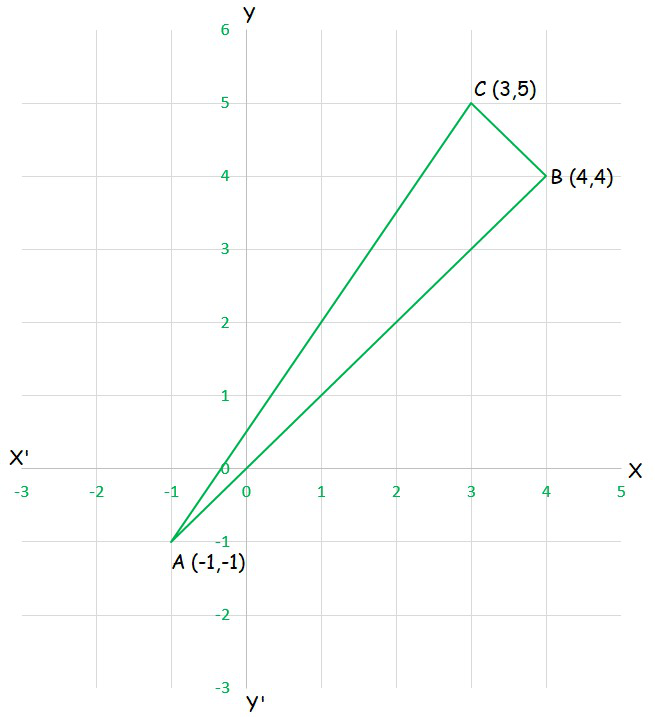As The slope ‘m’ of the line which is passing through the point (x1,y1) and (x2,y2) will be given as

m = (y2 – y1)/(x2 – x1) where, (x2 ≠ x1)

Let m1 be the slope of the line AB, then

m1 = (4 –(-1))/(4 –(-1)) = 1 ……………….(1)

Let m2 be the slope of the line BC, then

m2 = (3-4)/(5-4) = -1  …………………….(2)

Let m3 be the slope of the line AC, then

m3 = (5-(-1))/(3-(-1)) = 6/4 = 3/2  ………………..(3)

From (1) and (2), we can conclude

m1.m2 = -1.1 = -1

Hence, the lines AB and BC are perpendicular to each other.

Hence, given triangle is right-angled at B (4, 4)

### Question 7. Find the slope of the line, which makes an angle of 30° with the positive direction of y-axis measured anticlockwise.

Solution:

We know that if a line makes an angle of 30° with the positive direction of y-axis measured anti-clock-wise , then the angle made by the line with the positive direction of x- axis measure anti-clock-wise is

90° + 30° = 120°

∴ The slope of the given line is

tan(120°) = tan (180° – 60°)

= – tan 60°

= –√3

### Question 8. Find the value of x for which the points (x, – 1), (2,1) and (4, 5) are collinear.

Solution:

If the points (x, – 1), (2, 1) and (4, 5) are collinear, then

Slope of AB = Slope of BC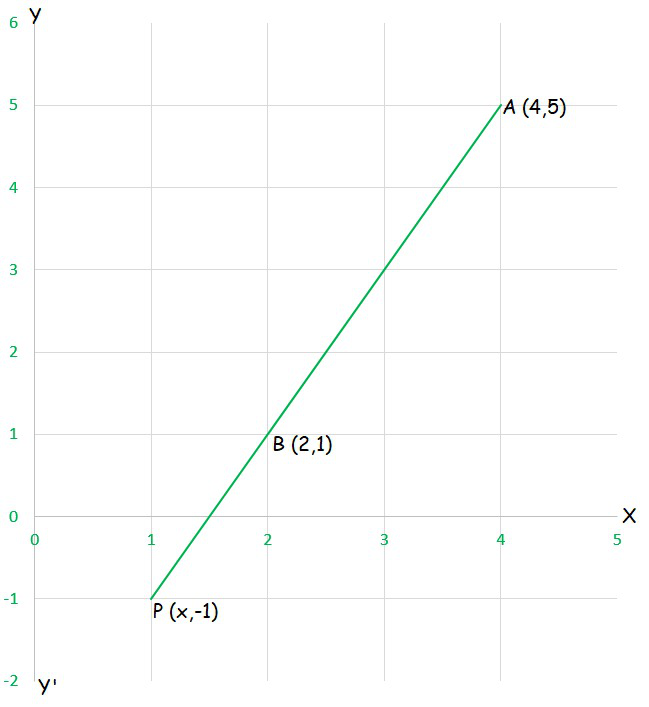Slope of AB = (1-(-1))/(2-x)

= 2/(2-x) ……………….(1)

Slope of BC = (5-1)/(4-2)

= 4/2

= 2  ………………..(2)

from (1) and (2)

2 = 2(2-x)

2 = 4 – 2x

2x = 4 – 2

2x = 2

x = 2/2

= 1

Hence, the required value of x is 1.

### Question 9. Without using the distance formula, show that points (– 2, – 1), (4, 0), (3, 3) and (–3, 2) are the vertices of a parallelogram.

Solution:

Let the given point be A (4, 0) , B ( 3, 3) C ( -3, 2) and D (-2, -1) of quadrilateral.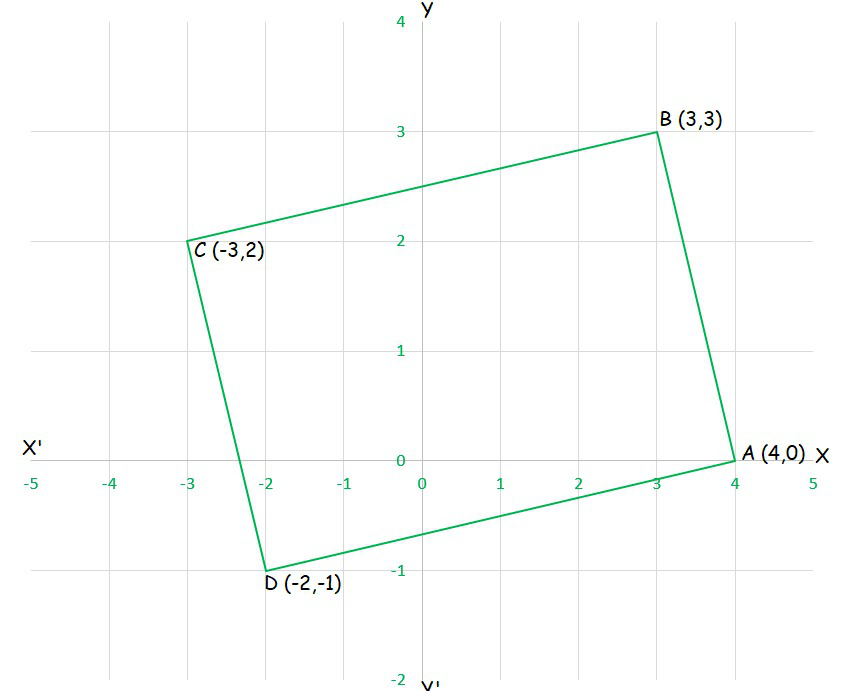So now, The slope of AD = (0-(-1))/(4-(-2)) = 1/6

The slope of BC = (3-2)/(3-(-3)) = 1/6

Hence, slope of AD = Slope of BC

Now,

The slope of AB = (3-0)/(3-4) = 3/-1 = -3

The slope of CD = (2-(-1))/(-3-(-2)) = 3/-1 = -3

Hence, slope of AB = Slope of CD

Hence, AB ∥ CD ……………………(2)

Hence, from (1) and (2), we can conclude that

The pair of opposite sides are quadrilateral are parallel, so we can say that ABCD is a parallelogram.

Hence the given vertices, A (4, 0), B (3, 3) C (-3, 2) and D (-2, -1) are vertices of a parallelogram.

### Question 10. Find the angle between the x-axis and the line joining the points (3,–1) and (4,–2).

Solution:

As The slope ‘m’ of the line which is passing through the point (x1,y1) and (x2,y2) will be given as

m = (y2 – y1)/(x2 – x1) where, (x2 ≠ x1)

So now, The slope ‘m’ of the line which is passing through the point (3,–1) and (4,–2) will be

m = (-2 –(-1))/(4-3)

= (-2+1)/(4-3)

= -1/1

= -1

as, m = tan θ

tan θ = -1

θ = (90° + 45°) = 135°

Hence, The angle between the x-axis and the line joining the points (3, –1) and (4, –2) is 135°.

### Question 11. The slope of a line is double of the slope of another line. If tangent of the angle between them is 1/3, find the slopes of the lines.

Solution:

Let us consider ‘m1’ and ‘m’ be the slope of the two given lines such that m1 = 2m and,

tan θ = 1/3

We know that if θ is the angle between the lines l1 and l2 with slope m1 and m2, then

tan θ = |(m2-m1) /(1+m1m2)|

so here,

1/3 = |(2m-m) /(1+2m2)|

1/3 = |m/(1+2m2)|

1/3 = ± (m/(1+2m2))

So taking, CASE 1

1/3 = + (m/(1+2m2))

2m2 – 3m + 1 = 0

2m2 – 2m – m + 1 = 0

2m (m – 1) – 1(m – 1) = 0

m = 1 or 1/2 ……………..(1)

And now, solving CASE 2

1/3 = – (m/(1+2m2))

1+2m2 = -3m

2m2 +1 +3m = 0

2m (m+1) + 1(m+1) = 0

(2m+1) (m+1)= 0

m = -1 or -1/2 ………………………(2)

So from (1) and (2) we can conclude that,

The pairs of slopes can be (1 and 2), (-1 and -2), (1 and 1/2) and (-1 and -1/2).

### k – y1 = m (h – x1)

Solution:

As it is given that, the slope of the line is ‘m’

The slope of the line passing through (x1, y1) and (h, k) is (k – y1)/(h – x1)

So,

m = (k – y1)/(h – x1)

(k – y1) = m (h – x1)

Hence, proved.

### Question 13. If three points (h, 0), (a, b) and (0, k) lie on a line, show that a/h + b/k = 1.

Solution:

If the given points A (h, 0), B (a, b) and C (0, k) lie on a line, then,

Slope of AB = slope of BC

(b – 0)/(a – h) = (k – b)/(0 – a)

By simplifying, we get

-ab = (k-b) (a-h)

-ab = ka- kh –ab +bh

ka +bh = kh

Divide both the sides by kh we get,

ka/kh + bh/kh = kh/kh

a/h + b/k = 1

Hence, proved.

### Question 14. Consider the following population and year graph (Fig 10.10), find the slope of the line AB and using it, find what will be the population in the year 2010?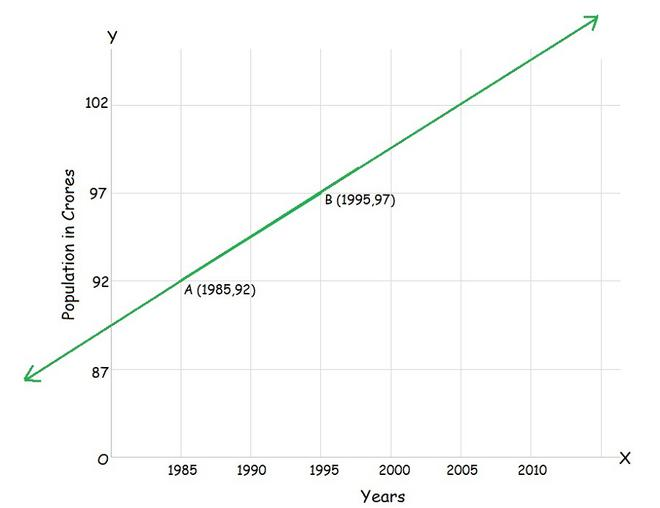Solution:

As The slope ‘m’ of the line which is passing through the point (x1,y1) and (x2,y2) will be given as

m = (y2 – y1)/(x2 – x1) where, (x2 ≠ x1)

So, the slope ‘m’ of the line which is passing through the point (1985,92) and (1995,97) will be given as

m = (97 – 92)/(1995 – 1985) = 5/10 = 1/2

Let ‘y’ be the population in the year 2010. Then, according to the given graph, AB must pass through point C (2010, y).

slope of AB = slope of BC

1/2 = (y-97)/(2010-1995)

15/2 = y – 97

y = 7.5 + 97 = 104.5

Hence, the slope of the line AB is 1/2, and

In the year 2010 the population will be 104.5 crores.

My Personal Notes arrow_drop_up
Related Tutorials